Latest Banking jobs   »

# Quantitative Aptitude Quiz For SBI Clerk Mains 2022- 17th December

Directions (1-3): Find the approximate value of (?) in the following questions.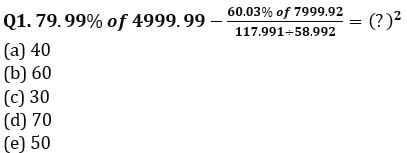Q2. 70.03% of 900.003-(34.991 of 16.02÷4.999)= ?+193.021
(a) 445
(b) 325
(c) 380
(d) 415
(e) 480

Q3. ((46.03% of 2999.98)÷68.996 of (10.01% of 20))= ?
(a) 50
(b) 40
(c) 20
(d) 30
(e) 10

Direction (4 – 5): There are a total of 24 fruits in a bag containing Mangoes, Oranges and Apple. Number of each type of fruit is different in the bag and difference between number of apples & number of mangoes is same as difference between number of mangoes & number of oranges in the bag. Probability of selecting one orange from the bag is greater than 0.20 and number of apples are maximum in the bag. (Mangoes > Oranges)

Q4. Number of oranges in the bag can be?
(A) 6
(B) 7
(C) 8
(D) 5
(E) 9
(a) Only option A, B, C possible
(b) Only option B, D & E possible
(c) Only option A, B, & D possible
(d) All of above options are possible
(e) Only option C, D & E possible

Q5. Find the maximum possible probability of selecting two mangoes from the bag?
(a) 7/69
(b) 7/64
(c) 7/72
(d) 7/82
(e) 7/81

Directions (6-10): In the following questions, two equations (I) and (II) are given. You have to solve both the equations and mark the appropriate answer.

Q6. I. 27x²-60x+32=0
II. 24y²-53y+28=0
(a) x>y
(b) x<y
(c) x≥y
(d) x≤y
(e) x=y or no relation.

Q7. I. (x-7)²=1
II. (y-9)²=y(1-y)+39
(a) x>y
(b) x<y
(c) x≥y
(d) x≤y
(e) x=y or no relation.

Q8. I. 15x²+46x+35=0
II. 24y²+59y+36=0
(a) x>y
(b) x<y
(c) x≥y
(d) x≤y
(e) x=y or no relation.

Q9. I. (x-3)²+(x-5)^2=x(x-4)-1
II. 6y²-53y+117=0
(a) x>y
(b) x<y
(c) x≥y
(d) x≤y
(e) x=y or no relation.

Q10. I. 20x²-61x+45=0
II. 8y²-26y+21=0
(a) x>y
(b) x<y
(c) x≥y
(d) x≤y
(e) x=y or no relation.

Directions (11-15): Read the given information carefully and answer the following questions. Line graph shows the percentage distribution of the employees in different departments in a firm.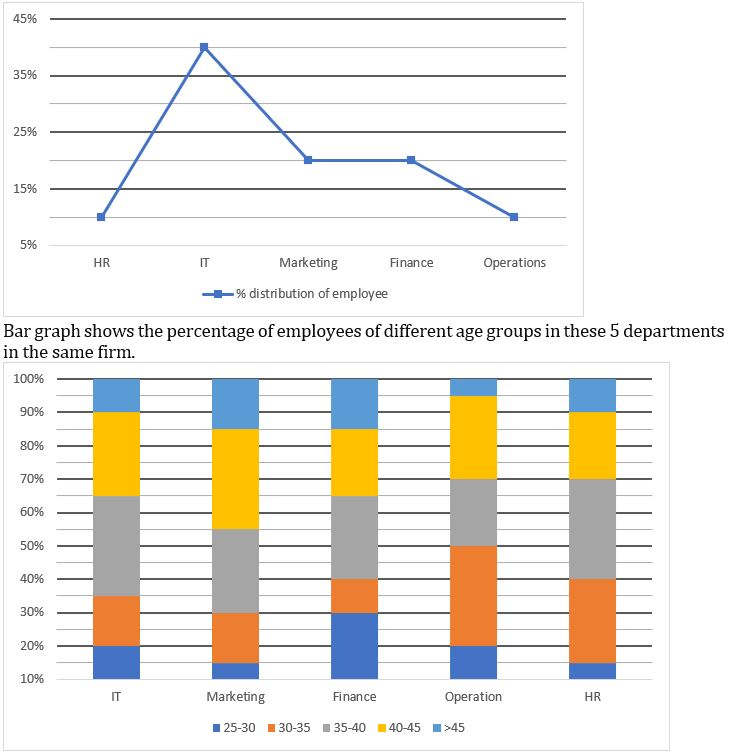Q11. The number of employees of age group 35 – 40 in the finance department is what percent more or less than the number of employees of age group 25 – 30 in the operations department?
(a) 175%
(b) 180%
(c) 120%
(d) 150%
(e) 125%

Q12. The total number of employees in the firm of age group more than 45 years from all the departments together form what percent of the total employees in the firm?
(a) 17.5%
(b) 18%
(c) 11.5%
(d) 15%
(e) 12.5%

Q13. The total number of employees in the IT department of age group 25 – 30 is 60. Find the total number of employees in the marketing department who belong to the age group of 40 – 45.?
(a) 35
(b) 45
(c) 40
(d) 30
(e) 50

Q14. If all the employees of age group more than 45 years from Finance department retire from their post and the same number of new employees join the same department but belong to the age group of 30 – 35 years, then find the number of employees in Finance department of age group 30 – 35 years, if the number of employees who retired is 150.
(a) 250
(b) 265
(c) 275
(d) 300
(e) 280

Q15. Find the average of employees in finance and HR, if the difference in the number of employees in IT department of age group more than 45 and the number of employees in the operations department of age group 40 – 45 is 45?
(a) 510
(b) 450
(c) 480
(d) 440
(e) 420

Solutions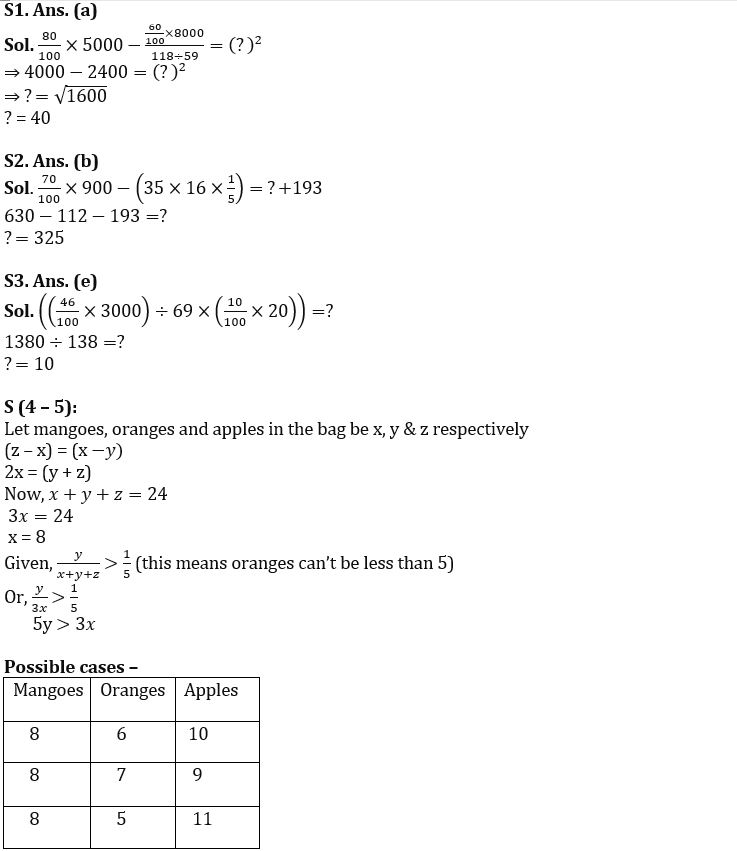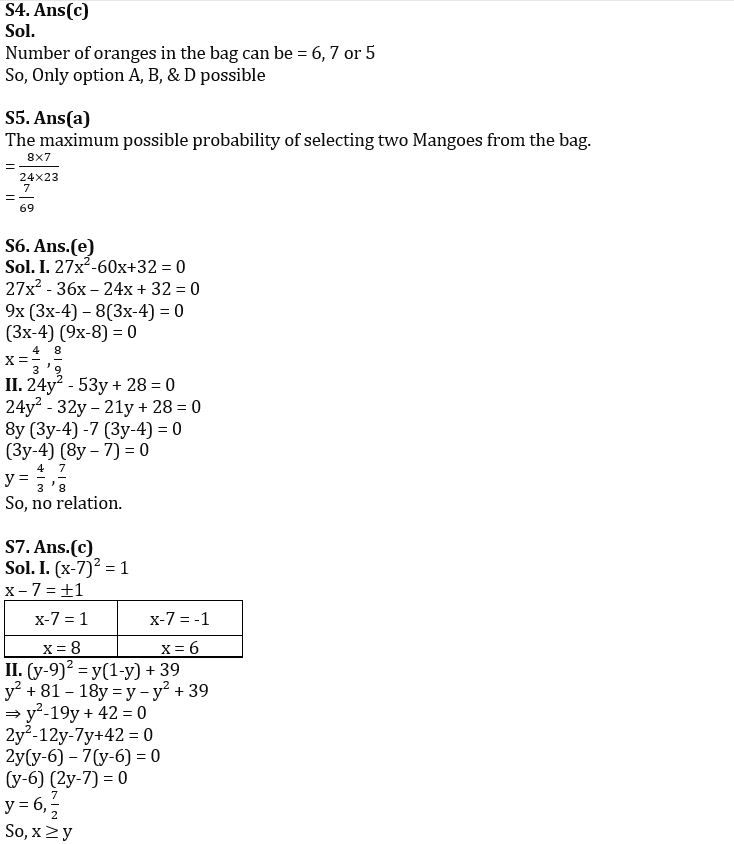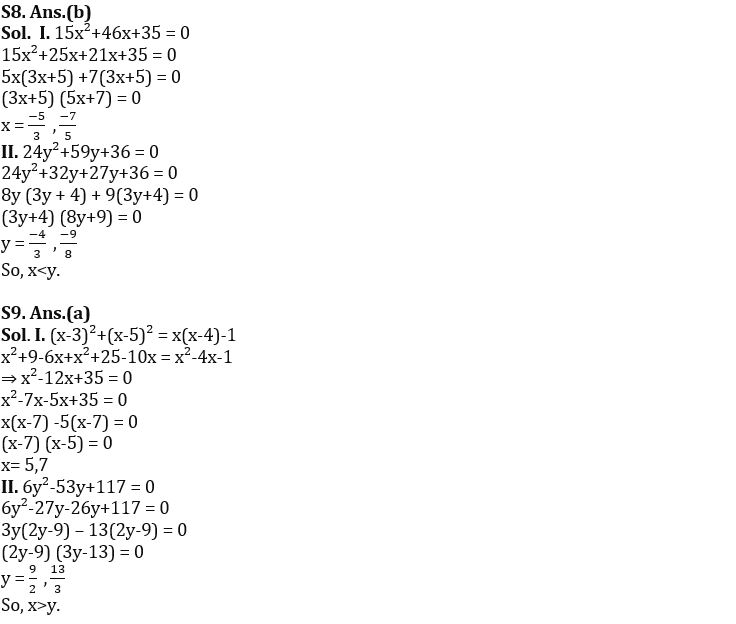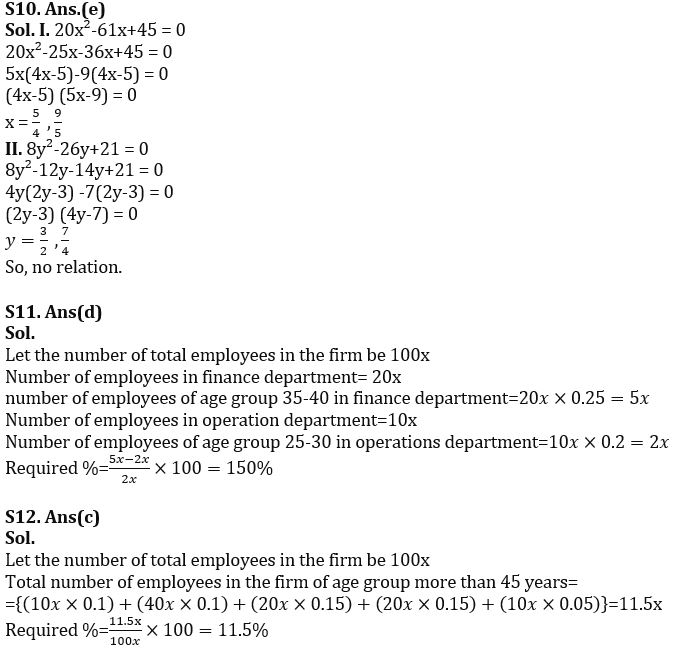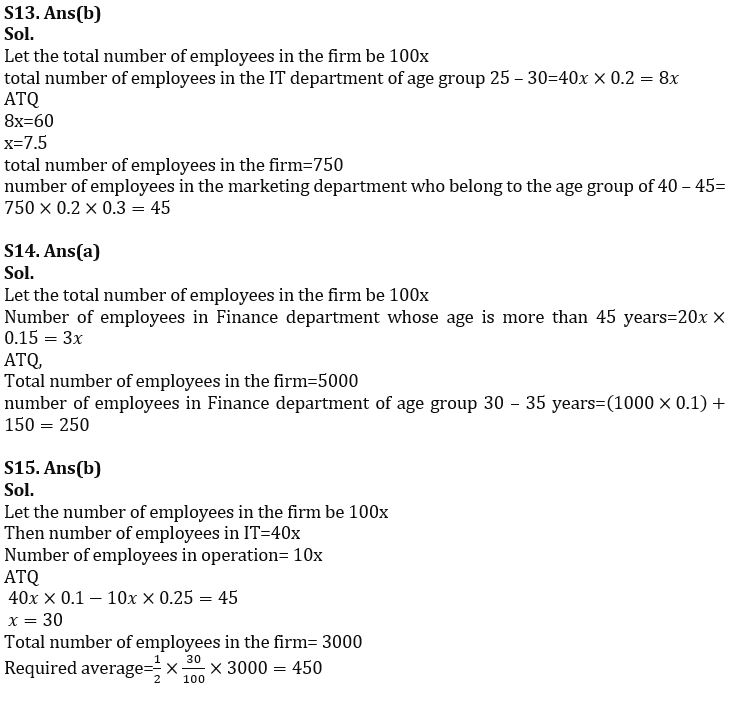#### Congratulations!Union Budget 2023-24: Free PDF